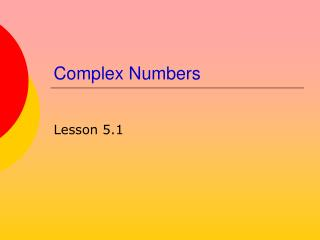DownloadDownload PresentationComplex Numbers

# Complex Numbers

Download Presentation## Complex Numbers

- - - - - - - - - - - - - - - - - - - - - - - - - - - E N D - - - - - - - - - - - - - - - - - - - - - - - - - - -
##### Presentation Transcript

1. Complex Numbers Lesson 5.1

2. It's any number you can imagine The Imaginary Number i • By definition • Consider powers if i

3. Using i • Now we can handle quantities that occasionally show up in mathematical solutions • What about

4. Imaginary part Real part Complex Numbers • Combine real numbers with imaginary numbers • a + bi • Examples

5. Try It Out • Write these complex numbers in standard form a + bi

6. Operations on Complex Numbers • Complex numbers can be combined with • addition • subtraction • multiplication • division • Consider

7. Operations on Complex Numbers • Division technique • Multiply numerator and denominator by the conjugate of the denominator

8. Complex Numbers on the Calculator • Possible result • Reset modeComplex formatto Rectangular • Now calculator does desired result

9. Complex Numbers on the Calculator • Operations with complex on calculator Make sure to use the correct character for i. Use 2nd-i

10. Warning • Consider • It is tempting to combine them • The multiplicative property of radicals only works for positive values under the radical sign • Instead use imaginary numbers

11. Try It Out • Use the correct principles to simplify the following:

12. Assignment • Lesson 5.1 • Page 340 • Exercises 1 – 69 EOO